PRINTABLE FOR KIDS

XII (12) HSC

XI (11) FYJC
X (10) SSC

### Practice Set 3.1 Arithmetic Progression Class 10th Mathematics Part 1 MHB Solution

Practice Set 3.1

1. 2, 4, 6, 8, . . . Which of the following sequences are A.P. ? If they are A.P. find…
2. 2 , 5/2 , 3 , 7/3 , Which of the following sequences are A.P. ? If they are A.P. find…
3. - 10, - 6, - 2, 2, . . . Which of the following sequences are A.P. ? If they are A.P.…
4. 0.3, 0.33, .0333, . . . Which of the following sequences are A.P. ? If they are A.P.…
5. 0, - 4, - 8, - 12, . . . Which of the following sequences are A.P. ? If they are A.P.…
6. - 1/5 , - 1/5 , - 1/5 , l Which of the following sequences are A.P. ? If they are A.P.…
7. 3 , 3 + root 2 , 3+2 root 2 , 3+3 root 2 , l Which of the following sequences are A.P.…
8. 127, 132, 137, . . . Which of the following sequences are A.P. ? If they are A.P. find…
9. a = 10, d = 5 Write an A.P. whose first term is a and common difference is d in each…
10. a = - 3, d = 0 Write an A.P. whose first term is a and common difference is d in each…
11. a = - 7 , d = 1/2 Write an A.P. whose first term is a and common difference is d in…
12. a = - 1.25, d = 3 Write an A.P. whose first term is a and common difference is d in…
13. a = 6, d = - 3 Write an A.P. whose first term is a and common difference is d in each…
14. a = - 19, d = - 4 Write an A.P. whose first term is a and common difference is d in…
15. 5, 1, - 3, - 7, . . . Find the first term and common difference for each of the A.P.…
16. 0.6, 0.9, 1.2, 1.5, . . . Find the first term and common difference for each of the…
17. 127, 135, 143, 151, . . . Find the first term and common difference for each of the…
18. 1/4 , 3/4 , 5/4 , 7/4 , l Find the first term and common difference for each of the…

###### Practice Set 3.1

Question 1.

Which of the following sequences are A.P. ? If they are A.P. find the common difference.

2, 4, 6, 8, . . .

2, 4, 6, 8, . . .

Here, the first term, a1 = 2

Second term, a2 = 4

a3 = 6

Now, common difference = a2 – a1 = 4 – 2 = 2

Also, a3 – a2 = 6 – 4 = 2

Since, the common difference is same.

Hence the terms are in Arithmetic progression with common difference, d = 2.

Question 2.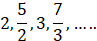Here, the first term, a1 = 2

Second term,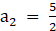Third Term, a3 = 3

Now, common difference =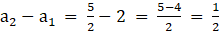Also,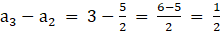Since, the common difference is same.

Hence the terms are in Arithmetic progression with common difference,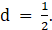Question 3.

Which of the following sequences are A.P. ? If they are A.P. find the common difference.

– 10, – 6, – 2, 2, . . .

– 10, – 6, – 2,2, . . .

Here, the first term, a1 = – 10

Second term, a2 = – 6

a3 = – 2

Now, common difference = a2 – a1 = – 6 – ( – 10) = – 6 + 10 = 4

Also, a3 – a2 = – 2 – ( – 6) = – 2 + 6 = 4

Since, the common difference is same.

Hence the terms are in Arithmetic progression with common difference, d = 4.

Question 4.

Which of the following sequences are A.P. ? If they are A.P. find the common difference.

0.3, 0.33, .0333, . . .

0.3, 0.33, 0.333,…..

Here, the first term, a1 = 0.3

Second term, a2 = 0.33

a3 = 0.333

Now, common difference = a2 – a1 = 0.33 – 0.3 = 0.03

Also, a3 – a2 = 0.333 – 0.33 = 0.003

Since, the common difference is not same.

Hence the terms are not in Arithmetic progression

Question 5.

Which of the following sequences are A.P. ? If they are A.P. find the common difference.

0, – 4, – 8, – 12, . . .

0, – 4, – 8, – 12, . . .

Here, the first term, a1 = 0

Second term, a2 = – 4

a3 = – 8

Now, common difference = a2 – a1 = – 4 – 0 = – 4

Also, a3 – a2 = – 8 – ( – 4) = – 8 + 4 = – 4

Since, the common difference is same.

Hence the terms are in Arithmetic progression with common difference, d = – 4.

Question 6.

Which of the following sequences are A.P. ? If they are A.P. find the common difference.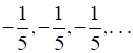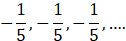Here, the first term,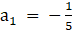Second term,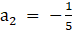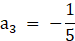Now, common difference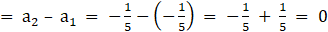Also,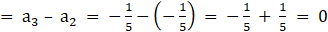Since, the common difference is same.

Hence the terms are in Arithmetic progression with common difference,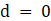.

Question 7.

Which of the following sequences are A.P. ? If they are A.P. find the common difference.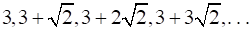3, 3 + √2, 3 + 2√2, 3 + 3√2, ….

Here, the first term, a1 = 3

Second term, a2 = 3 + √2

a3 = 3 + 2√2

Now, common difference = a2 – a1 = 3 + √2 – 3 = √2

Also, a3 – a2 = 3 + 2√2 –(3 + √2) = 3 + 2√2 – 3 – √2 = √2

Since, the common difference is same.

Hence the terms are in Arithmetic progression with common difference, d = √2 .

Question 8.

Which of the following sequences are A.P. ? If they are A.P. find the common difference.

127, 132, 137, . . .

127, 132, 137, . . .

Here, the first term, a1 = 127

Second term, a2 = 132

a3 = 137

Now, common difference = a2 – a1 = 132 – 127 = 5

Also, a3 – a2 = 137 – 132 = 5

Since, the common difference is same.

Hence the terms are in Arithmetic progression with common difference, d = 5.

Question 9.

Write an A.P. whose first term is a and common difference is d in each of the following.

a = 10, d = 5

a = 10, d = 5

Let a1 = a = 10

Since, the common difference d = 5

Using formula an + 1 = an + d

Thus, a2 = a1 + d = 10 + 5 = 15

a3 = a2 + d = 15 + 5 = 20

a4 = a3 + d = 20 + 5 = 25

Hence, An A.P with common difference 5 is 10, 15, 20, 25,….

Question 10.

Write an A.P. whose first term is a and common difference is d in each of the following.

a = – 3, d = 0

a = – 3, d = 0

Let a1 = a = – 3

Since, the common difference d = 0

Using formula an + 1 = an + d

Thus, a2 = a1 + d = – 3 + 0 = – 3

a3 = a2 + d = – 3 + 0 = – 3

a4 = a3 + d = – 3 + 0 = – 3

Hence, An A.P with common difference 0 is – 3, – 3, – 3, – 3,….

Question 11.

Write an A.P. whose first term is a and common difference is d in each of the following.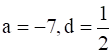a = – 7,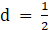Let a1 = a = – 7

Since, the common differenceUsing formula an + 1 = an + d

Thus,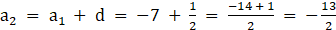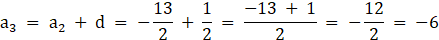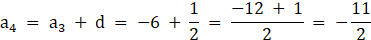Hence, An A.P with common differenceis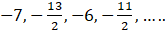Question 12.

Write an A.P. whose first term is a and common difference is d in each of the following.

a = – 1.25, d = 3

a = – 1.25, d = 3

Let a1 = a = – 1.25

Since, the common difference d = 3

Using formula an + 1 = an + d

Thus, a2 = a1 + d = – 1.25 + 3 = 1.75

a3 = a2 + d = 1.75 + 3 = 4.75

a4 = a3 + d = 4.75 + 3 = 7.75

Hence, An A.P with common difference 3 is – 1.25, 1.75, 4.75, 7.75

Question 13.

Write an A.P. whose first term is a and common difference is d in each of the following.

a = 6, d = – 3

a = 6, d = – 3

Let a1 = a = 6

Since, the common difference d = – 3

Using formula an + 1 = an + d

Thus, a2 = a1 + d = 6 + ( – 3) = 6 – 3 = 3

a3 = a2 + d = 3 + ( – 3) = 3 – 3 = 0

a4 = a3 + d = 0 + ( – 3) = – 3

Hence, An A.P with common difference – 3 is 6, 3, 0, – 3…

Question 14.

Write an A.P. whose first term is a and common difference is d in each of the following.

a = – 19, d = – 4

a = – 19, d = – 4

Let a1 = a = – 19

Since, the common difference d = – 4

Using formula an + 1 = an + d

Thus, a2 = a1 + d = – 19 + ( – 4) = – 19 – 4 = – 23

a3 = a2 + d = – 23 + ( – 4) = – 23 – 4 = – 27

a4 = a3 + d = – 27 + ( – 4) = – 27 – 4 = – 31

Hence, An A.P with common difference – 4 is – 19, – 23, – 27, – 31,….

Question 15.

Find the first term and common difference for each of the A.P.

5, 1, – 3, – 7, . . .

5, 1, – 3, – 7, . . .

First term a1 = 5

Second term a2 = 1

Third term a3 = – 3

We know that d = an + 1 – an

Thus, d = a2 – a1 = 1 – 5 = – 4

Hence, the common difference d = – 4 and first term is 5

Question 16.

Find the first term and common difference for each of the A.P.

0.6, 0.9, 1.2, 1.5, . . .

0.6, 0.9, 1.2, 1.5, . . .

First term a1 = 0.6

Second term a2 = 0.9

Third term a3 = 1.2

We know that d = an + 1 – an

Thus, d = a2 – a1 = 0.9 – 0.6 = 0.3

Hence, the common difference d = 0.3 and first term is 0.6

Question 17.

Find the first term and common difference for each of the A.P.

127, 135, 143, 151, . . .

127, 135, 143, 151, . . .

First term a1 = 127

Second term a2 = 135

Third term a3 = 143

We know that d = an + 1 – an

Thus, d = a2 – a1 = 135 – 127 = 8

Hence, the common difference d = 8 and first term is 127

Question 18.

Find the first term and common difference for each of the A.P.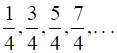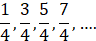First term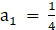Second term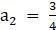Third term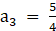We know that d = an + 1 – an

Thus,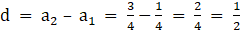Hence, the common differenceand first term is## PDF FILE TO YOUR EMAIL IMMEDIATELY PURCHASE NOTES & PAPER SOLUTION. @ Rs. 50/- each (GST extra)

SUBJECTS

HINDI ENTIRE PAPER SOLUTION

MARATHI PAPER SOLUTION
SSC MATHS I PAPER SOLUTION
SSC MATHS II PAPER SOLUTION
SSC SCIENCE I PAPER SOLUTION
SSC SCIENCE II PAPER SOLUTION
SSC ENGLISH PAPER SOLUTION
SSC & HSC ENGLISH WRITING SKILL
HSC ACCOUNTS NOTES
HSC OCM NOTES
HSC ECONOMICS NOTES
HSC SECRETARIAL PRACTICE NOTES

2019 Board Paper Solution

HSC ENGLISH SET A 2019 21st February, 2019

HSC ENGLISH SET B 2019 21st February, 2019

HSC ENGLISH SET C 2019 21st February, 2019

HSC ENGLISH SET D 2019 21st February, 2019

SECRETARIAL PRACTICE (S.P) 2019 25th February, 2019

HSC XII PHYSICS 2019 25th February, 2019

CHEMISTRY XII HSC SOLUTION 27th, February, 2019

OCM PAPER SOLUTION 2019 27th, February, 2019

HSC MATHS PAPER SOLUTION COMMERCE, 2nd March, 2019

HSC MATHS PAPER SOLUTION SCIENCE 2nd, March, 2019

SSC ENGLISH STD 10 5TH MARCH, 2019.

HSC XII ACCOUNTS 2019 6th March, 2019

HSC XII BIOLOGY 2019 6TH March, 2019

HSC XII ECONOMICS 9Th March 2019

SSC Maths I March 2019 Solution 10th Standard11th, March, 2019

SSC MATHS II MARCH 2019 SOLUTION 10TH STD.13th March, 2019

SSC SCIENCE I MARCH 2019 SOLUTION 10TH STD. 15th March, 2019.

SSC SCIENCE II MARCH 2019 SOLUTION 10TH STD. 18th March, 2019.

SSC SOCIAL SCIENCE I MARCH 2019 SOLUTION20th March, 2019

SSC SOCIAL SCIENCE II MARCH 2019 SOLUTION, 22nd March, 2019

XII CBSE - BOARD - MARCH - 2019 ENGLISH - QP + SOLUTIONS, 2nd March, 2019

HSC Maharashtra Board Papers 2020

(Std 12th English Medium)

HSC ECONOMICS MARCH 2020

HSC OCM MARCH 2020

HSC ACCOUNTS MARCH 2020

HSC S.P. MARCH 2020

HSC ENGLISH MARCH 2020

HSC HINDI MARCH 2020

HSC MARATHI MARCH 2020

HSC MATHS MARCH 2020

SSC Maharashtra Board Papers 2020

(Std 10th English Medium)

English MARCH 2020

HindI MARCH 2020

Hindi (Composite) MARCH 2020

Marathi MARCH 2020

Mathematics (Paper 1) MARCH 2020

Mathematics (Paper 2) MARCH 2020

Sanskrit MARCH 2020

Important-formula

THANKS